# Introduction

R-package VFP is based on the ideas of the standalone software Variance Function Program by William A. Sadler [https://www.aacb.asn.au/resources/useful-tools/variance-function-program-v16]. This is also the reason why we chose to name it VFP instead of e.g. RPP for R Precision Profiles or similar.

Precision profiles are a comprehensive and intuitive way to visualize variability over a part of or the entire measuring range of a measuring method. Manufacturers of IVD-assays need to state the precision of their products in the method sheet (package insert). This is usually done in tabular format presenting total (im)precision and individual variance components at given concentrations of samples used in the respective precision experiment. The units of variability reported are either standard deviation (SD) and/ or coefficient of variation (CV) which is a percentage in regard to the sample concentration. The drawback of using tables is obvious. Variability of any concentration in between two reported samples and not close to one of these is not immediately clear.

Precision performance table as usually included in package insert.
Mean Reproducibility %CV Between-Site %CV Between-Day %CV Repeatability %CV
sample.P1 12.08 8.6 5.1 3.5 6.0
sample.P2 41.58 4.4 3.1 0.8 3.1
sample.Q3 55.75 4.1 3.2 1.3 2.2
sample.Q4 165.70 3.8 3.3 0.8 1.7
sample.P5 379.10 2.4 1.3 0.5 2.0
sample.Q6 414.30 3.7 3.1 0.4 2.1

Additionally, any variability estimated is carrying more or less error in the same sense as data points in a regression analysis are not all located on the regression line. Precision profiles model the non-linear relationship between sample mean and variance taking into account the information provided by multiple precision-experiments. Below, an example is given, showing the precision profile on CV-scale for reproducibility variability of the CA19_9 data set from R-package VCA (2nd column in table above):

##
## Model 4  ... finished.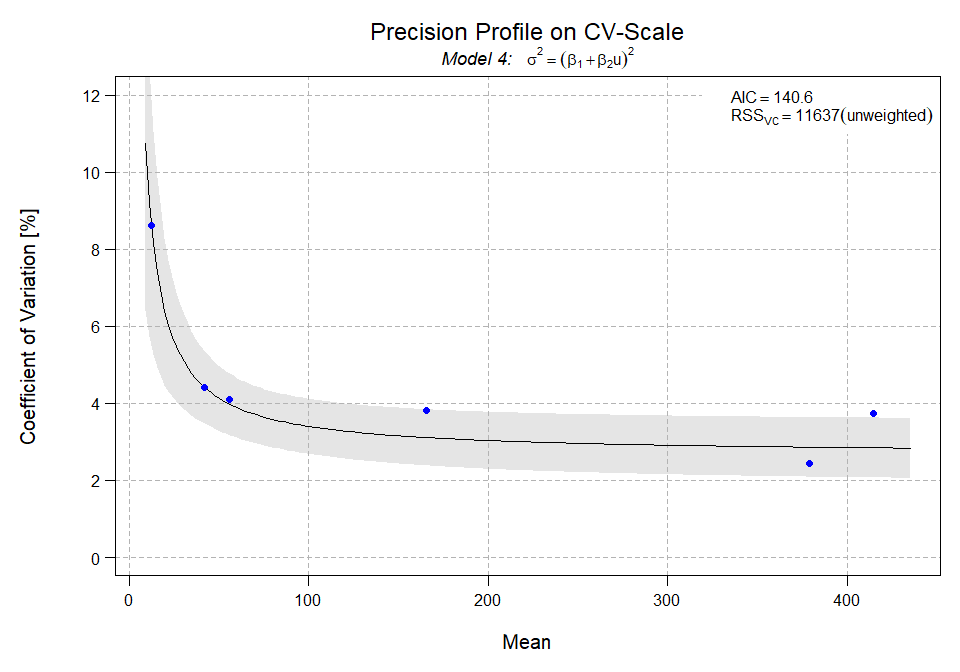Each point in this plot corresponds to the reproducibility on CV[%] scale of one sample, i.e. there were six samples measured at three sites, on 5 days per site, and 5 replicated measurements per day. This data set corresponds to the numerical example of the CLSI EP05-A3 guideline showing a typical form of a precision profile with larger relative variability for lower concentrations and an almost constant %CV for larger concentrations. The precision profile for this data is based on model 4, i.e. for this non-linear function the Akaike Information Criterion ($$-2 \times logLik + 2 \times p$$, p being the number of parameters) takes the lowest number. These are provided by R-package gnm which we chose for performing the actual model fitting.

# How To Generate a Precision Profile

Any precision profile is based on the outcome of a precision experiment. The required input for fitting one of the models provided is Mean, Variance and the Degrees of Freedom for multiple samples used in the precision experiment. Let’s start with performing the analysis of such a precision experiment.

library(VFP)
library(VCA)
# CLSI EP05-A3 example data
data(CA19_9)

Now perform the variance component analysis (VCA) for each sample using batch-processing. Here, we the anovaVCA function from R-package VCA is used.

VCA.CA19_9 <- anovaVCA(result~site/day, CA19_9, by="sample")

The result will be a list of VCA-objects.

class(VCA.CA19_9)
##  "list"
sapply(VCA.CA19_9, class)
## sample.P1 sample.P2 sample.Q3 sample.Q4 sample.P5 sample.Q6
##     "VCA"     "VCA"     "VCA"     "VCA"     "VCA"     "VCA"
print(VCA.CA19_9[], digits=4)
##
##
## Result Variance Component Analysis:
## -----------------------------------
##
##   Name     DF      SS      MS      VC     %Total  SD     CV[%]
## 1 total    11.3181                 1.0869 100     1.0425 8.6292
## 2 site     2       22.0419 11.0209 0.3843 35.3578 0.6199 5.1312
## 3 site:day 12      16.964  1.4137  0.1778 16.3565 0.4216 3.4899
## 4 error    60      31.488  0.5248  0.5248 48.2857 0.7244 5.9963
##
## Mean: 12.0813 (N = 75)
##
## Experimental Design: balanced  |  Method: ANOVA

Each VCA-object stores the information for one sample and there are multiple types of variability which can be used to construct a precision profile. Total variability corresponds to reproducibility in this example. One can also use repeatability (error) which is the pure assay imprecision. If one decides to use the sum of repeatability and day-to-day (aka between-day, here “site:day”) one uses intermediate precision or within-lab variability. The next code snippet shows how this information can be extracted from the list of VCA-objects.

Function getMat.VCA does the job. Without selecting a specific element, i.e. variance component or sequence of these, total variability will be used by default.

mat.total <- getMat.VCA(VCA.CA19_9)
print(mat.total)
##                Mean        DF         VC
## sample.P1  12.08133 11.318142   1.086864
## sample.P2  41.58400  7.604586   3.376848
## sample.Q3  55.74667  4.896189   5.257296
## sample.Q4 165.65600  3.331477  39.752635
## sample.P5 379.09067 16.709246  85.059893
## sample.Q6 414.28667  4.112871 241.089499

Note:
This input does not need to be derived from a variance component analysis performed with R-package VCA. One can can easily compile the required input from externally performed analyses as well.

To extract repeatability one can either specify “error” or the index of this variance component which will be 4 here.

mat.rep <- getMat.VCA(VCA.CA19_9, "error")
print(mat.rep)
##                Mean DF        VC
## sample.P1  12.08133 60  0.524800
## sample.P2  41.58400 60  1.634800
## sample.Q3  55.74667 60  1.559933
## sample.Q4 165.65600 60  7.812800
## sample.P5 379.09067 60 56.966933
## sample.Q6 414.28667 60 73.959000

In this example Intermediate Precision consists of two variance components, repeatability and day-to-day. To select these specify the sequence of indexes. Note, that this will only be possible for models that were fitted using ANOVA-estimation, thus, any model fitted using REML will result in an error.

mat.ip <- getMat.VCA(VCA.CA19_9, 3:4)
print(mat.ip)
##                Mean       DF         VC
## sample.P1  12.08133 51.42153  0.7025733
## sample.P2  41.58400 68.08386  1.7579600
## sample.Q3  55.74667 51.60925  2.0831067
## sample.Q4 165.65600 57.44560  9.6790933
## sample.P5 379.09067 69.14653 60.1530533
## sample.Q6 414.28667 69.89176 76.9797867

The next step towards a precision profile consists of fitting a VFP-model. This can be done for a single model or in batch-mode. The latter means instead of fitting one model after another, one can select a set of models. There are 10 models implemented in R-package VFP:

1. $$\sigma^2$$, constant variance
2. $$\sigma^2=\beta_1 \times u^2$$, constant CV
3. $$\sigma^2=\beta_1 + \beta_2 \times u^2$$, mixed constant, proportional variance
4. $$\sigma^2=(\beta_1 + \beta_2 \times u)^K$$, constrained power model, constant exponent
5. $$\sigma^2=\beta_1+\beta_2 \times u^K$$, alternative constrained power model
6. $$\sigma^2=\beta_1 + \beta_2 \times u + \beta_3 \times u^J$$, unconstrained power model for variance functions with a minimum
7. $$\sigma^2=\beta_1 + \beta_2 \times u^J$$, alternative unconstrained power model
8. $$\sigma^2=(\beta_1 + \beta_2 \times u)^J$$, unconstrained power model (default model of W. Sadler)
9. $$\sigma^2=\beta_1 \times u^J$$, similar to CLSI EP17 model
10. $$CV=\beta_1 \times u^J$$, exact CLSI EP17 model (fitted by linear regression on logarithmic scale)

Fitting all ten models is somehow redundant if constant K is chosen to be equal to 2, since models 3 and 5 are equivalent and these are constrained versions of model 7 where the exponent is also estimated. The latter also applies to model 4 which is a constrained version of model 8. Nevertheless, since computation time is not critical here for typical precision-profiles (of in-vitro diagnostics precision experiments) we chose to offer batch-processing as well.

During computation, all models are internally re-parameterized so as to guarantee that the variance function is positive in the range of u from 0 to $$u_{max}$$. In models 7 and 8, J is restricted to $$0.1 < J < 10$$ to avoid the appearance of sharp hooks. Occasionally, these restrictions may lead to a failure of convergence. This is then a sign that the model parameters are on the boundary and that the model fits the data very badly. This should not be taken as reason for concern. It occurs frequently for model 6 when the variance function has no minimum, which is normally the case.

If batch-processing has been performed, one can select one of the models or use the best fitting model automatically if none is specified (see below) in all subsequent function calls on the returned VFP-object.

Below model 1 is fitted to the total variability data.

 tot.m1 <- fit.vfp(mat.total, model.no=1)
##
## Model 1  ... finished.

The standard print-method for VCA-objects is identical to the summary-method yielding:

tot.m1
##
##
## (VFP) Variance-Function
## -----------------------
##
## Model 1:     sigma^2=beta1
##
## Coefficients:
## beta1
## 54.39
##
## AIC = 232.6  RSS = 43870  Deviance = 64.31 GoF P-value= 0.04715

Now, all ten available models are fitted to the total variability data in batch mode. Note, model 5 is skipped since it is identical to model 3 without changing the default setting K=2 to something different.

 tot.all <- fit.vfp(mat.total, 1:10)
##
## Model 1  ... finished.
## Model 2  ... finished.
## Model 3  ... finished.
## Model 4  ... finished.
## Model 5  ... skipped!
## Model 6  ... finished.
## Model 7  ... finished.
## Model 8  ... finished.
## Model 9  ... finished.
## Model 10 ... finished.
 # 'summary' presents more details for multi-model objects
summary(tot.all)
##
##
##  +++ Summary Fitted VFP-Model(s) +++
## -------------------------------------
##
## Call:
## fit.vfp(Data = mat.total, model.no = 1:10)
##
##  [+++ 9 Models Fitted +++]
##
## Model_1:  sigma^2=beta1
## Model_2:  sigma^2=beta1*u^2
## Model_3:  sigma^2=beta1+beta2*u^2
## Model_4:  sigma^2=(beta1+beta2*u)^2
## Model_6:  sigma^2=beta1+beta2*u+beta3*u^J
## Model_7:  sigma^2=beta1+beta2*u^J
## Model_8:  sigma^2=(beta1+beta2*u)^J
## Model_9:  sigma^2=beta1*u^J
## Model_10:  cv=beta1*u^J
##
##
##  Model_1  Model_2  Model_3  Model_4  Model_6  Model_7  Model_8  Model_9
##    43870   136876     9334    11637    12833    12824    12677    15284
## Model_10
##    17364
##
##  [+++ AIC +++]
##
##  Model_1  Model_2  Model_3  Model_4  Model_6  Model_7  Model_8  Model_9
##    232.6    201.9    149.6    140.6    143.1    141.1    141.6    149.5
## Model_10
##    439.7
##
##  [+++ Deviance +++]
##
##  Model_1  Model_2  Model_3  Model_4  Model_6  Model_7  Model_8  Model_9
##   64.310   22.790    2.712    1.875    1.757    1.758    1.799    2.699
## Model_10
##  351.800
##
##  [+++ Best Model +++]
##
## Model 4:     sigma^2=(beta1+beta2*u)^2
##
## Coefficients:
##   beta1   beta2
## 0.73290 0.02671
##
## AIC = 140.6  RSS = 11637  Deviance = 1.875  GoF P-value= 1.000
##
##  [+++ Note +++]
##
## Model 5 will not be fitted because it is identical to model 3 with 'K=2'!

# Plotting Precision Profiles

Our recommendation is to always fit all models and using the best one. Of course, if two fitted models are very similar in their AIC one can use the less complex model. The fitted model(s) is/are stored in a VFP-object. Calling the plot-method for these objects will always generate a precision profile on variance scale (VC), which is the scale model fitting takes place on.

# not selecting a specific model automatically chooses the one with lowest AIC
plot(tot.all)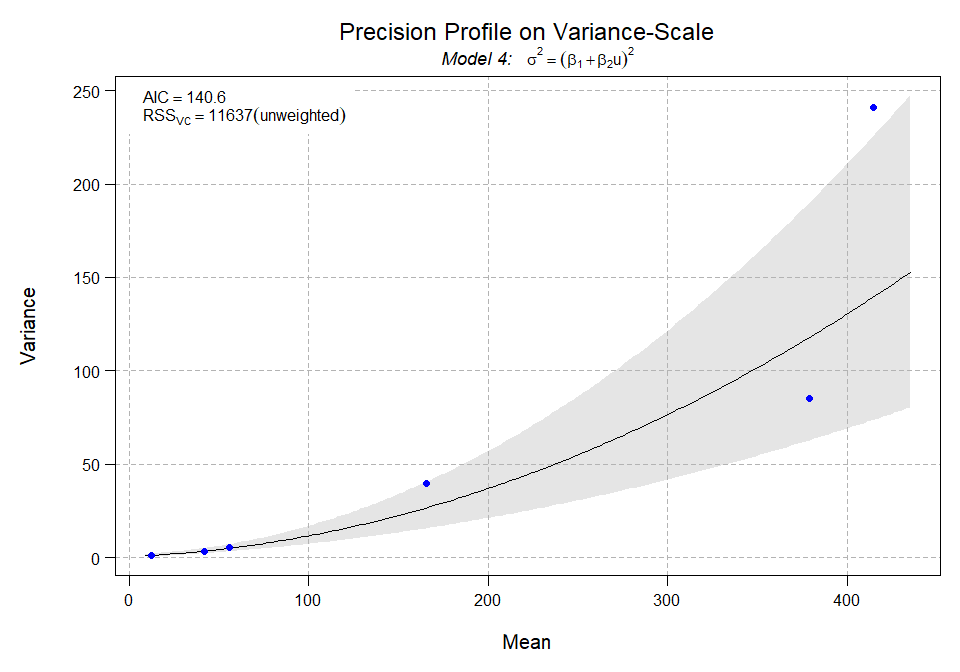One can plot the precision profile on variance (type=“vc”, default), SD (type=“sd”) or CV (type=“cv”) scale.

# selecting one specific model is easy
plot(tot.all, model.no=7, type="cv", ylim=c(0, 10))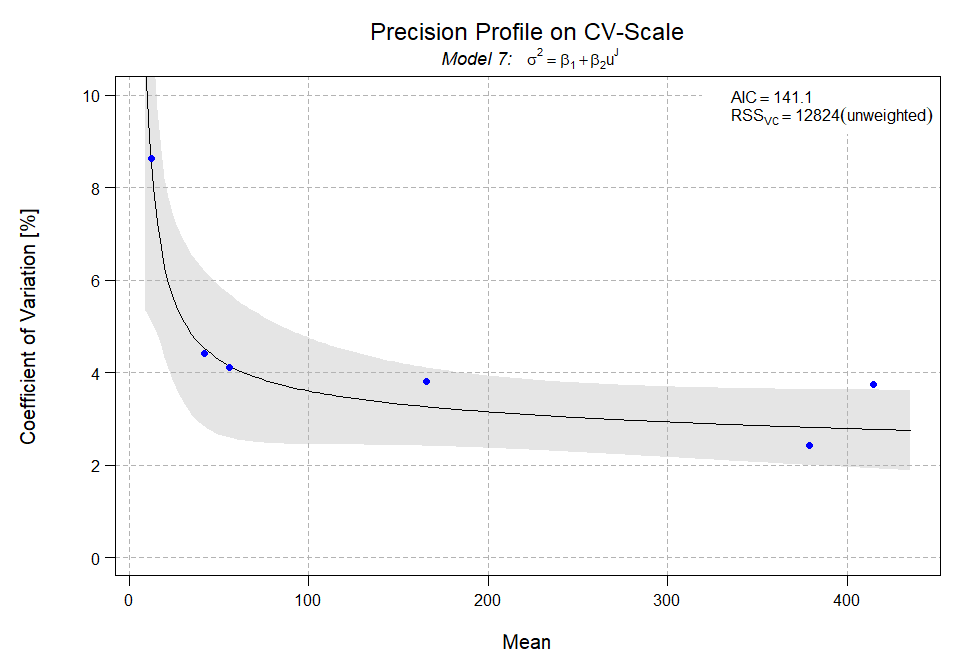There are plenty of options to customize the visual appearance of a precision profile. One can add multiple precision profiles to one plot as done in the CLSI EP05-A3 guideline from which this example is borrowed. Below, we start with plotting the fitted precision profile for reproducibility, where concentrations on the X-axis are plotted on log-scale. Here, we also leave some space in the right margin to add a legend later on.

plot(   tot.all, model.no=8, mar=c(5.1, 5, 4.1, 8),
type="cv", ci.type="none", Model=FALSE,
Line=list(col="blue", lwd=3),
Points=list(pch=15, col="blue", cex=1.5),
xlim=c(10, 450), ylim=c(0,10),
Xlabel=list(text="CA19-9, kU/L (LogScale) - 3 Patient Pools, 3 QC Materials",
cex=1.25), Title=NULL,
Ylabel=list(text="% CV", cex=1.25, line=3),
Grid=NULL, Crit=NULL, log="x")

# We now add the precision profile for intermediate precision
# to the existing plot (add=TRUE).

mod8.ip <- fit.vfp(mat.ip, 8)
##
## Model 8  ... finished.
plot(mod8.ip, type="cv", add=TRUE, ci.type="none",
Points=list(pch=16, col="deepskyblue", cex=1.5),
Line=list(col="deepskyblue", lwd=3), log="x")

# Now, add the precision profile of repeatability.

mod8.rep <- fit.vfp(mat.rep, 8)
##
## Model 8  ... finished.
plot(mod8.rep, type="cv", add=TRUE, ci.type="none",
Points=list(pch=17, col="darkorchid3", cex=1.5),
Line=list(col="darkorchid3", lwd=3), log="x")

# Finally, add a legend in the right margin.

legend.rm( x="center", pch=15:17, col=c("blue", "deepskyblue", "darkorchid3"),
cex=1, legend=c("Reproducibility", "Within-Lab", "Repeatability"),
box.lty=0)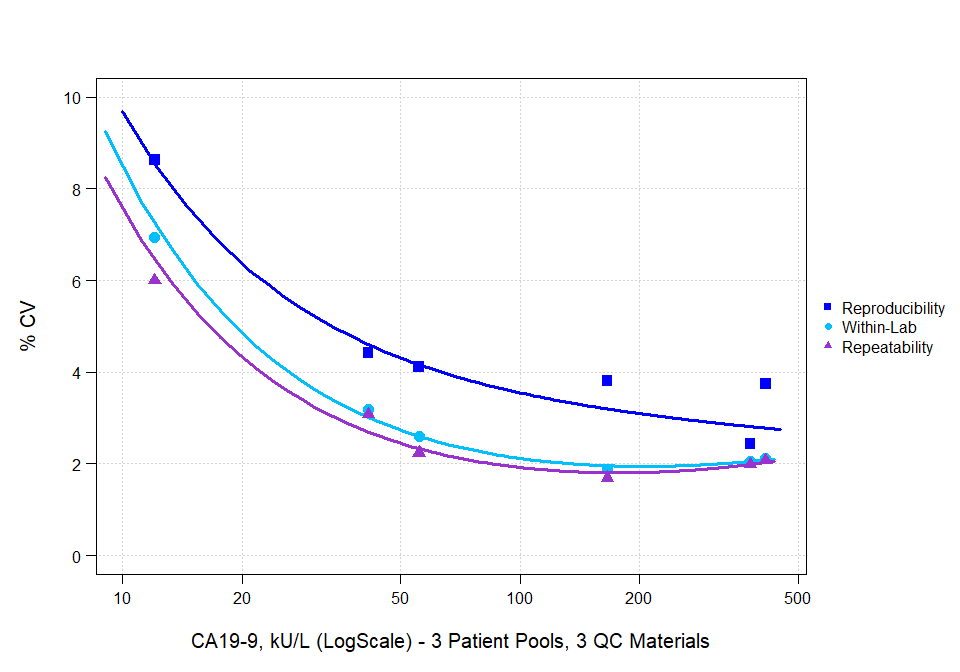We now conclude with an example showing many of the different features of the standard plot-method for VFP-objects.

plot(mod8.rep, BG="darkgray",
Points=list(pch=17, cex=1.5, col="blue"), Line=list(col="blue"),
Grid=list(x=seq(0, 450, 50), y=seq(0, 110, 10), col="white"),
Xlabel=list(cex=1.5, text="CA19-9 [U/mL]", col="blue"),
Ylabel=list(cex=1.5, text="Repeatability on Variance-Scale", col="blue"),
Crit=NULL)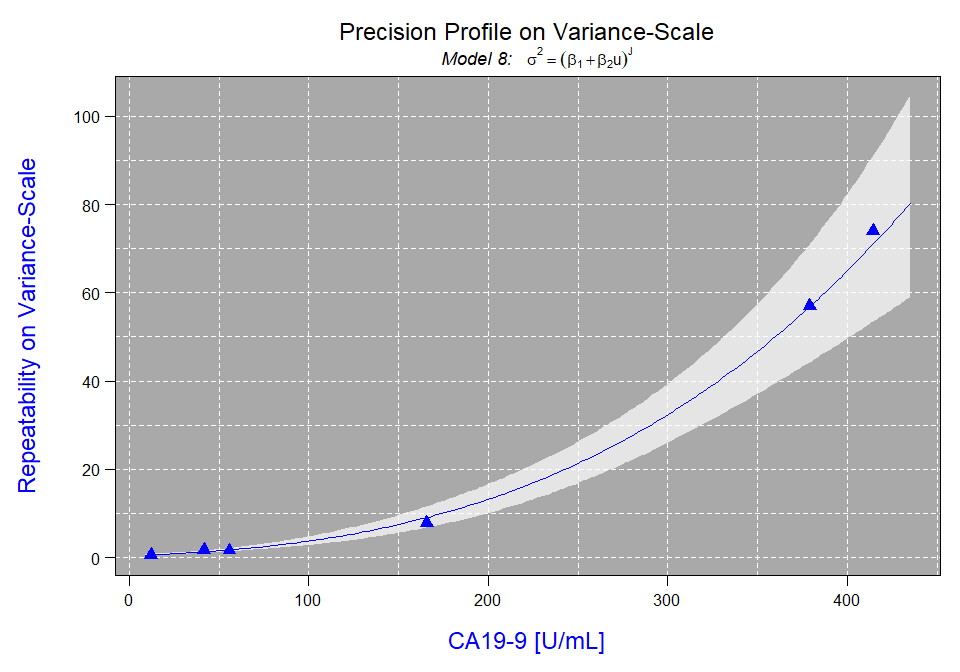# Functional Sensitivity and More

Beyond beautification options available in the plot-method for VFP-objects there is more useful functionality integrated. Actually, the functionality of functions predict.VFP and predictMean are integrated via argument Prediction in plot.VFP().
In the introduction, we talked about some drawbacks of presenting precision data in tables. Usually, one is interested in the (im)precision at a specific concentration, e.g. at a medical decision point. This can easily be inferred from a precision profile and visualized in one step using the Prediction argument. This can be used in multiple ways as shown below.

If a number or a vector of numbers is assigned to argument Prediction this will be interpreted as having specified Prediction=list(y=x), where x is the number. This will internally trigger calling function predictMean to derive that concentration at which the specified variability is reached. Note, that the scale of the Y-axis is here taken into account, i.e. if on CV-scale specifications mean percent CV which differs from SD- or variance-scales.
The main use-case for this is determining functional sensitivity of an assay, i.e. a concentrations at which e.g. a pre-specified CV is not exceeded for all concentrations larger than this value.

plot(mod8.rep, type="cv", ylim=c(0, 8), Prediction=5)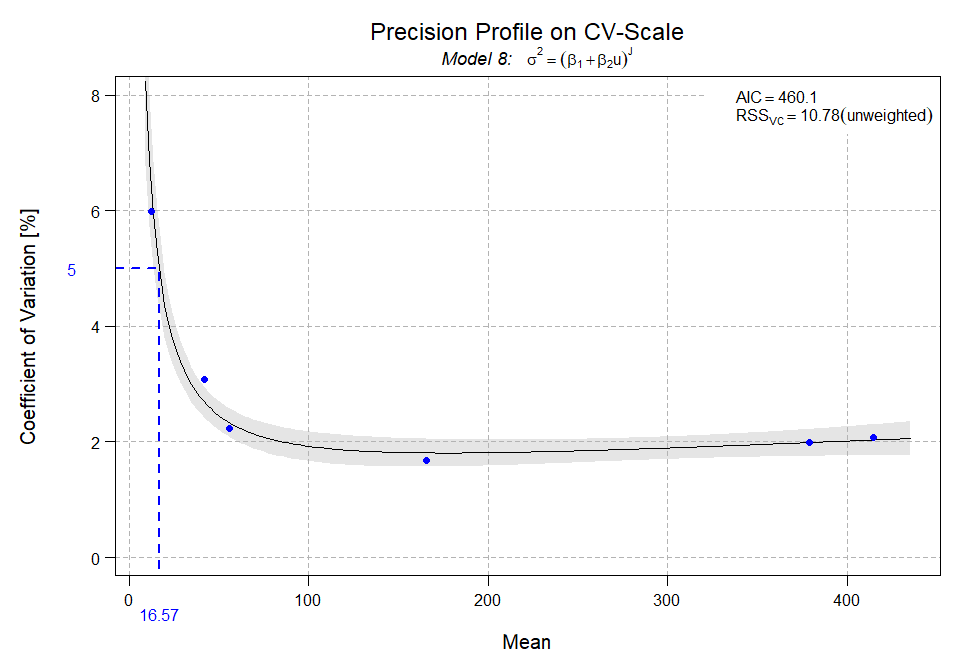To add confidence intervals, one can set argument Pred.CI=TRUE.

plot(mod8.rep, type="cv", ylim=c(0, 8), xlim=c(0, 100), Prediction=5, Pred.CI=TRUE)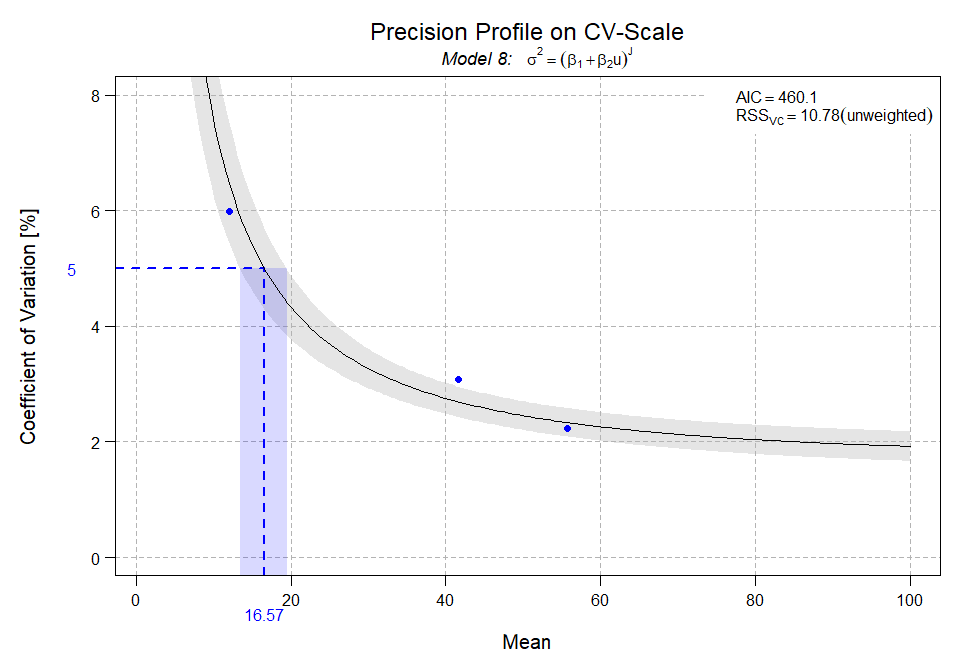Calling function predict.VFP within the plotting function can be achieved as shown below.

plot(mod8.rep, type="cv", ylim=c(0, 8), xlim=c(0, 100), Prediction=list(x=50), Pred.CI=TRUE)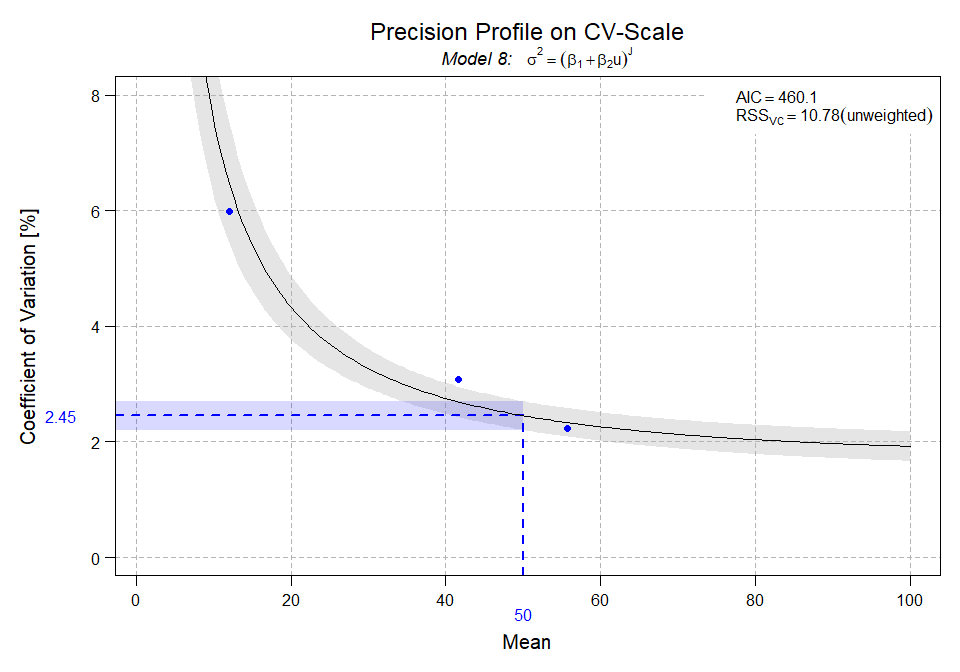Once a precision profile for an IVD-assay is established one can infer variability at any concentration of interest from it. It nicely summarizes the precision performance of an assay in a simple plot providing much higher information density than e.g. a simple table. One can also use it to derive functional sensitivity, which is specific to an assay, being that concentration at which a pre-defined variability is undercut for all concentrations larger than this value.

# C5 and C95 for Qualitative Tests

Another extremely useful property of precision profiles, predominantly required for qualitative tests, is to derive C5 and C95 concentrations. For qualitative tests, where an internal continuous response (ICR) is available from which the qualitative result is derived, e.g. negative or positive, non-reactive or. reactive, etc., precision profiles should be used to establish C5 and C95 according to the CLSI EP12 guideline. These concentrations correspond to samples which, when measured many times, are expected to yield proportions of measurements found having the condition of interest. In case of C5 this proportion is 5%, in case of C95 95% of the replicates are expected to have it. There is a function available in R-package VFP designed to provide this information based on a precision-profile stored as a VFP-object.

Assume values on the X-axis to be measurements of the ICR, which is usually available for manufacturers of qualitative IVD-assays.

deriveCx(mod8.rep, cutoff=40, start=35, Cx=0.05)
##        Mean          SD    Actual.p
## 38.22302704  1.08032254  0.04999995

The actual nice feature of this function is to visualize concentration Cx if requested via plot=TRUE.

deriveCx(mod8.rep, cutoff=40, start=35, Cx=0.05, plot=TRUE)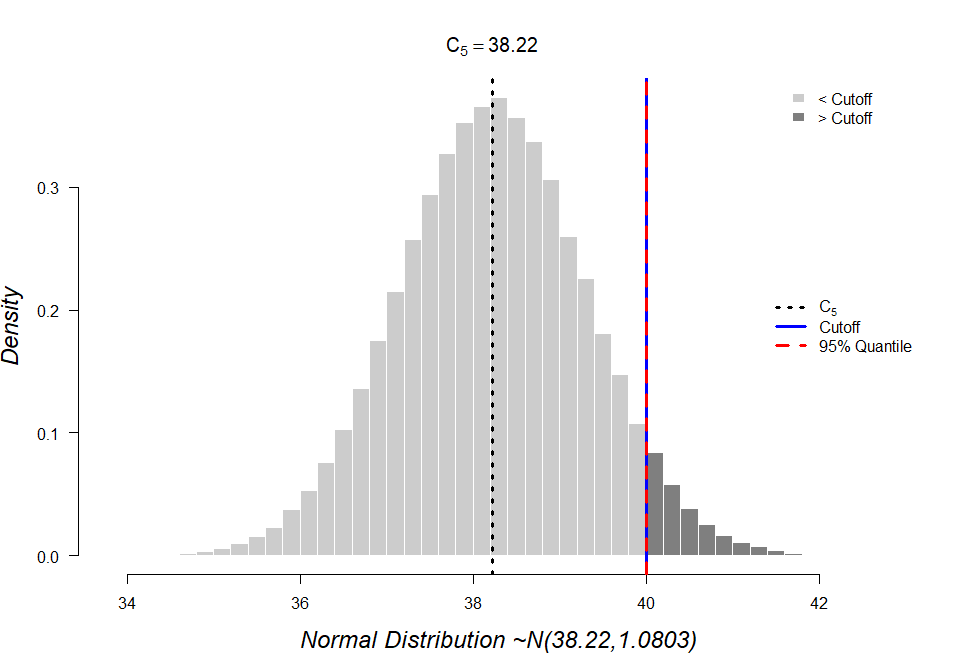##        Mean          SD    Actual.p
## 38.22302704  1.08032254  0.04999995

The same can be done for C95.

deriveCx(mod8.rep, cutoff=40, start=35, Cx=0.95, plot=TRUE)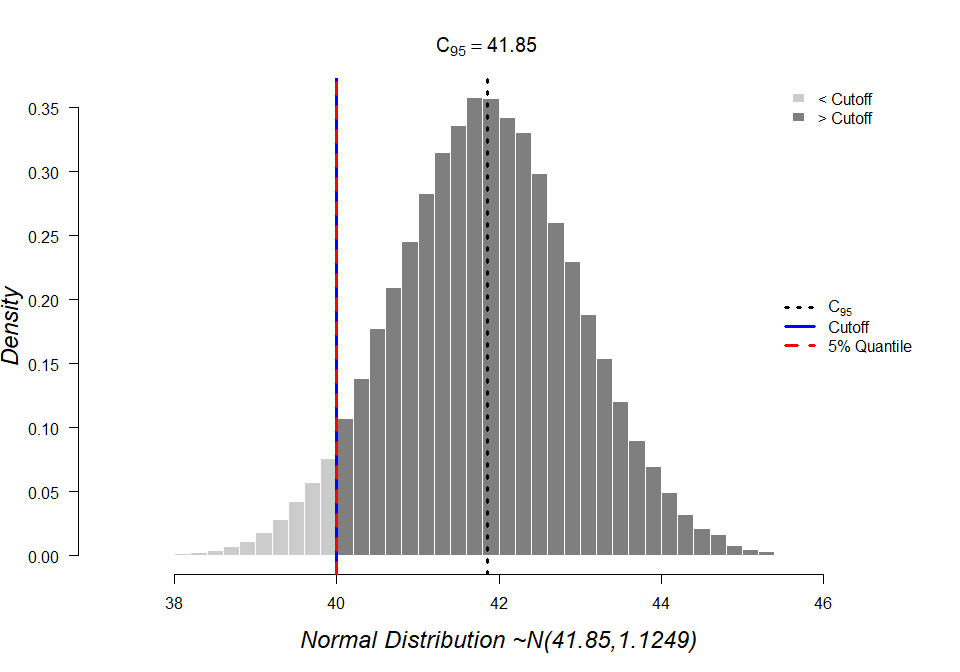##       Mean         SD   Actual.p
## 41.8503153  1.1249122  0.9499999

Now assume there are two cutoffs defining an equivocal zone in between, i.e. a re-test zone, gray zone or borderline results. Using function precisionPlot helps to nicely summarize all information linked to C5 and C95.

res <- precisionPlot(mod8.rep, cutoff=c(35, 40), prob=c(.05, .95), alpha2=.5)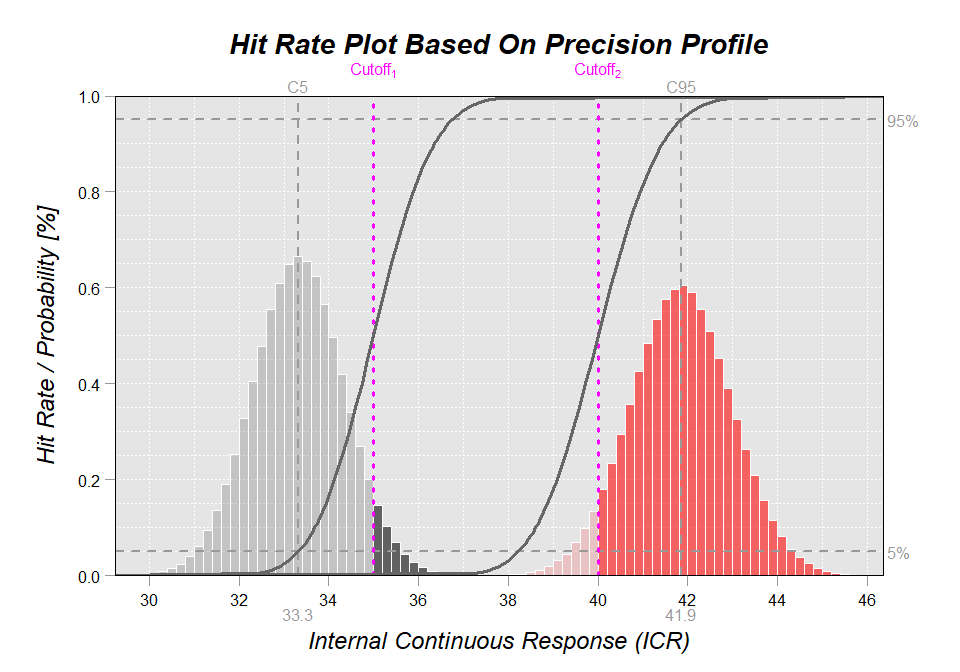Note, that this function invisibly returns computed results, which might be of interest.

print(str(res))
## List of 2
##  $cutoff1: num [1:41, 1:5] 0.005 0.01 0.02 0.03 0.04 0.05 0.06 0.07 0.08 0.09 ... ## ..- attr(*, "dimnames")=List of 2 ## .. ..$ : NULL
##   .. ..$: chr [1:5] "Cx" "Mean" "SD" "Actual.p" ... ##$ cutoff2: num [1:41, 1:4] 0.005 0.01 0.02 0.03 0.04 0.05 0.06 0.07 0.08 0.09 ...
##   ..- attr(*, "dimnames")=List of 2
##   .. ..$: NULL ## .. ..$ : chr [1:4] "Cx" "Mean" "SD" "Actual.p"
## NULL
head(res[])
##         Cx     Mean       SD   Actual.p Actual.p.rounded
## [1,] 0.005 32.39818 1.010092 0.00500001              0.5
## [2,] 0.010 32.64338 1.013014 0.01000002              1.0
## [3,] 0.020 32.91292 1.016229 0.02000003              2.0
## [4,] 0.030 33.08482 1.018282 0.03000004              3.0
## [5,] 0.040 33.21459 1.019832 0.04000005              4.0
## [6,] 0.050 33.32044 1.021098 0.05000005              5.0